# High School Math : How to find the angle of clock hands

## Example Questions

### Example Question #1 : How To Find The Angle Of Clock Hands

Find the angle between the minute and hour hand at 8:20 PM.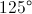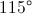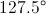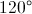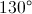Explanation:

The distance between each notch on the clock is 6 degrees because there are 360 degrees on the clock, and there are 60 notches total. The minute hand is at notch #20, and so it is 120 degrees from the top. The hour hand is a little past notch #40 because the time is a little past hour 8. Thus, the hour hand is a little past 240 degrees from the top, going clockwise (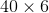degrees).

In each hour, the hour hand goes 5 notches, or 30 degrees.  Because it is now 20 minutes past the hour, a third of an hour has passed.  One third of 30 degrees is 10 degrees.  Thus, the hour hand is 10 degrees past notch #40.  The hour hand is 250 degrees from the top, going clockwise.  The angle between the two hands is thus 130 degrees.

### Example Question #2 : How To Find The Angle Of Clock Hands

When making a pie chart, how many degrees should be allotted for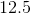percent?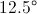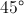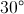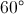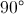Explanation:

This is a proportion problem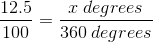so there are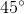inpercent of a circle

### Example Question #1 : How To Find The Angle Of Clock Hands

If it is 2:00 PM, what is the measure of the angle between the minute and hour hands of the clock?

30 degrees

60 degrees

90 degrees

120 degrees

45 degrees

60 degrees

Explanation:

First note that a clock is a circle made of 360 degrees, and that each number represents an angle and the separation between them is 360/12 = 30. And at 2:00, the minute hand is on the 12 and the hour hand is on the 2.  The correct answer is 2 * 30 = 60 degrees.

### Example Question #3 : How To Find The Angle Of Clock Hands

A clock currently reads 2:00. What is the size of the angle formed between the hour and minute hands?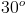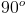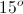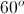Explanation:

The interior angle of a sector is equal the the angle of the sector. If the entire circumference was the sector, it would equal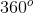. Additionally, if it were 12:00, the angle would be equal to. We can solve the problem by setting up a proportion. 2:00 will be two-twelfths of the circle past the 12:00 mark, and will be at an angle of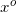.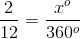Cross multiply and solve for.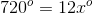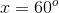### Example Question #3 : How To Find The Angle Of Clock Hands

It is 4 o’clock.  What is the measure of the angle formed between the hour hand and the minute hand?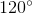Explanation:

At four o’clock the minute hand is on the 12 and the hour hand is on the 4.  The angle formed is 4/12 of the total number of degrees in a circle, 360.

4/12 * 360 = 120 degrees

### Example Question #1 : How To Find The Angle Of Clock Hands

If a clock reads 8:15 PM, what angle do the hands make?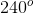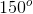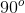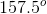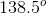Explanation:

A clock is a circle, and a circle always contains 360 degrees. Since there are 60 minutes on a clock, each minute mark is 6 degrees.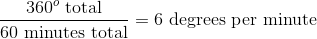The minute hand on the clock will point at 15 minutes, allowing us to calculate it's position on the circle.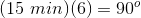Since there are 12 hours on the clock, each hour mark is 30 degrees.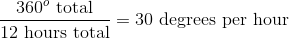We can calculate where the hour hand will be at 8:00.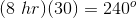However, the hour hand will actually be between the 8 and the 9, since we are looking at 8:15 rather than an absolute hour mark. 15 minutes is equal to one-fourth of an hour. Use the same equation to find the additional position of the hour hand.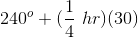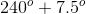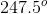We are looking for the angle between the two hands of the clock. The will be equal to the difference between the two angle measures.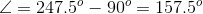### Example Question #1 : How To Find The Angle Of Clock Hands

What is the measure of the smaller angle formed by the hands of an analog watch if the hour hand is on the 10 and the minute hand is on the 2?

90°

56°

45°

120°

30°

120°

Explanation:

A analog clock is divided up into 12 sectors, based on the numbers 1–12. One sector represents 30 degrees (360/12 = 30). If the hour hand is directly on the 10, and the minute hand is on the 2, that means there are 4 sectors of 30 degrees between then, thus they are 120 degrees apart (30 * 4 = 120).

### Example Question #1 : How To Find The Angle Of Clock Hands

What is the measure, in degrees, of the acute angle formed by the hands of a 12-hour clock that reads exactly 3:10?

55°

60°

72°

35°

65°